机器学习笔记（十）EM算法及实践（以混合高斯模型（GMM）为例来次完整的EM）

====================================================================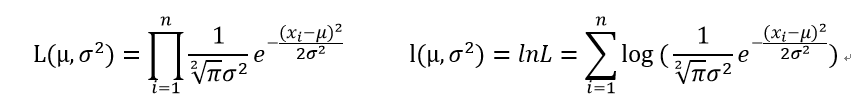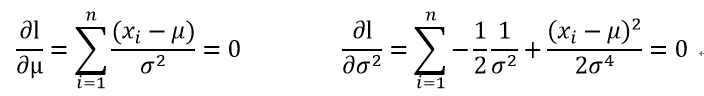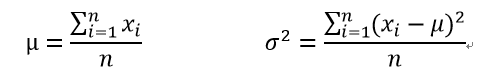====================================================================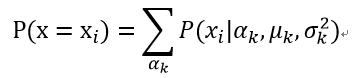Alpha代表了该样本属于某一人种的比例，其实就是隐藏的中间变量。Muk和sigmak^2为各类高斯分布的参数。按照我们上面的套路就是求对数似然概率再求导得到参数的估计，那么先来看看似然函数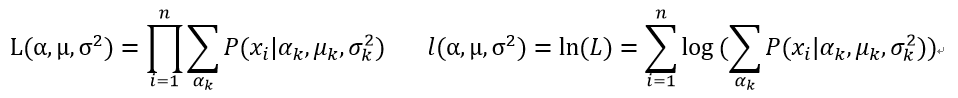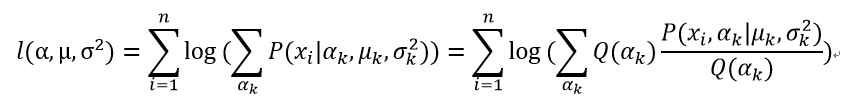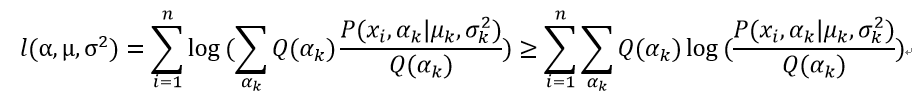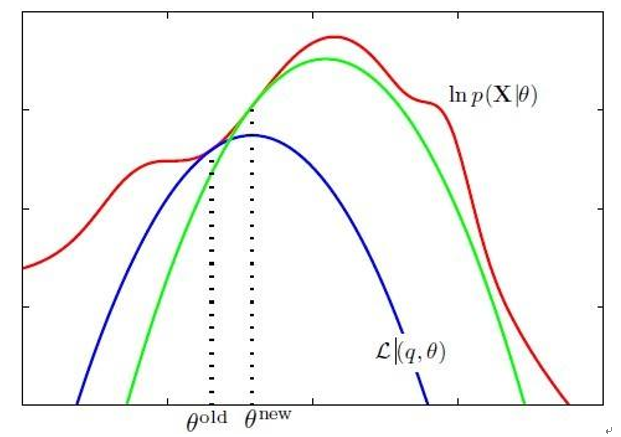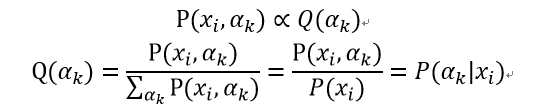1 拿到所有的观测样本，根据先验或者喜好先给一个参数估计。

2 根据这个参数估计和样本计算类别分布Q，得到最贴近对数似然函数的下界函数。

3 对下界函数求极值，更新参数分布。

4 迭代计算，直至收敛。

====================================================================高斯混合模型（GMM）

E-step 求最佳的类别分布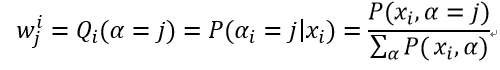M-step 更新参数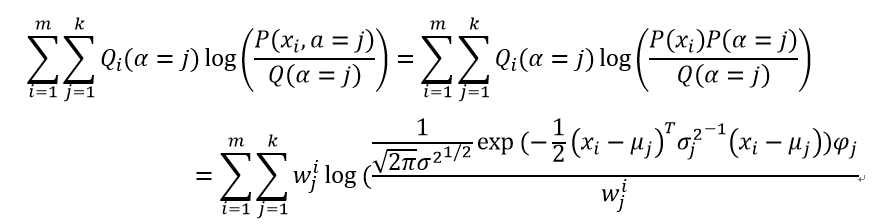Log函数里面全是乘积项这是我们最喜欢的形式，这样求导的时候但凡不相关的我们直接扔掉就行，待求参数mu,sigma^2,psi，依次求导为0就成。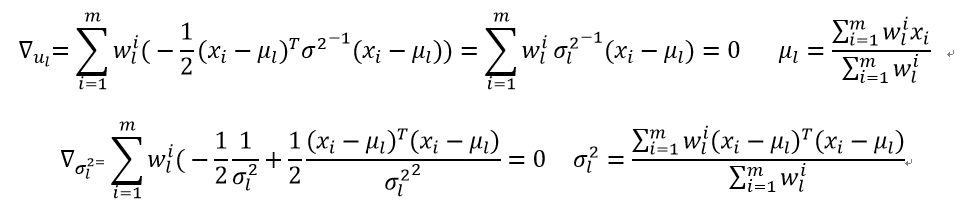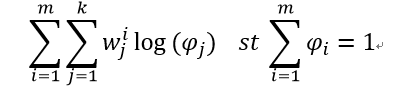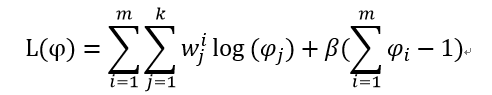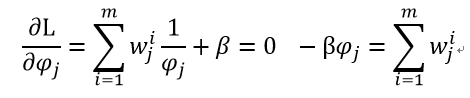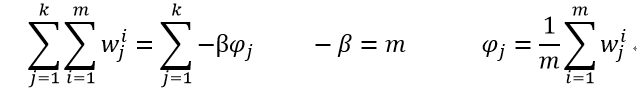1 给参数取初始值，开始迭代。

2 求每个样本对每个类别的概率，科学的叫法叫求响应度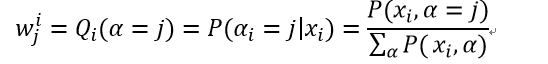3 更新模型参数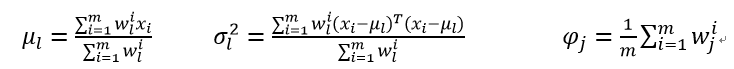4 重复23两步直至收敛。

====================================================================

import numpy as np
from sklearn import datasets
from sklearn.cluster import KMeans
from sklearn.mixture import GaussianMixture
#读取数据
x=iris.data[:,:2]
y=iris.target
mu = np.array([np.mean(x[y == i], axis=0) for i in range(3)])
print '实际均值 = \n', mu
#K-Means
kmeans=KMeans(n_clusters=3,init='k-means++',random_state=0)
y_hat1=kmeans.fit_predict(x)
mu1=np.array([np.mean(x[y_hat1 == i], axis=0) for i in range(3)])
print 'K-Means均值 = \n', mu1
print '分类正确率为',np.mean(y_hat1==y)
gmm=GaussianMixture(n_components=3,covariance_type='full', random_state=0)
gmm.fit(x)
print 'GMM均值 = \n', gmm.means_
y_hat2=gmm.predict(x)
print '分类正确率为',np.mean(y_hat2==y)


[[5.006  3.418]

[5.936  2.77 ]

[6.588  2.974]]

K-Means均值 =

[[5.77358491  2.69245283]

[ 5.006      3.418     ]

[ 6.81276596 3.07446809]]

GMM均值 =

[[5.01494511  3.44040237]

[ 6.69225795 3.03018616]

[ 5.90652226 2.74740414]]

import numpy as np
from sklearn import datasets
from sklearn.cluster import KMeans
from sklearn.mixture import GaussianMixture
#读取数据
x=iris.data[:,:2]
y=iris.target
mu = np.array([np.mean(x[y == i], axis=0) for i in range(3)])
print '实际均值 = \n', mu
#K-Means
kmeans=KMeans(n_clusters=3,init='k-means++',random_state=0)
y_hat1=kmeans.fit_predict(x)
y_hat1[y_hat1==0]=3
y_hat1[y_hat1==1]=0
y_hat1[y_hat1==3]=1
mu1=np.array([np.mean(x[y_hat1 == i], axis=0) for i in range(3)])
print 'K-Means均值 = \n', mu1
print '分类正确率为',np.mean(y_hat1==y)
gmm=GaussianMixture(n_components=3,covariance_type='full', random_state=0)
gmm.fit(x)
print 'GMM均值 = \n', gmm.means_
y_hat2=gmm.predict(x)
y_hat2[y_hat2==1]=3
y_hat2[y_hat2==2]=1
y_hat2[y_hat2==3]=2
print '分类正确率为',np.mean(y_hat2==y)


[[5.006  3.418]

[ 5.936 2.77 ]

[ 6.588 2.974]]

K-Means均值 =

[[5.006       3.418     ]

[ 5.77358491 2.69245283]

[ 6.81276596 3.07446809]]

GMM均值 =

[[5.01494511  3.44040237]

[ 6.69225795 3.03018616]

[ 5.90652226 2.74740414]]